# Tank

To cuboid tank whose bottom has dimensions of 9 m and 15 m were flow 1080 hectoliters of water. This was filled 40% of the tank volume. Calculate the depth of the tank.

h =  2 m

### Step-by-step explanation: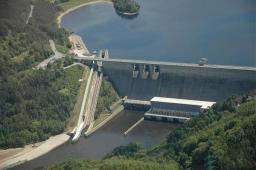Did you find an error or inaccuracy? Feel free to write us. Thank you!Tips to related online calculators
Do you know the volume and unit volume, and want to convert volume units?

## Related math problems and questions:

• Water tankA 288 hectoliter of water was poured into the tank with dimensions 12 m and 6 m bottom and 2 m depth. What part of the volume of the tank water occupied? Calculate the surface of tank wetted with water.
• Water tankWater tank shape of cuboid has dimensions of the bottom 7.5 meters and 3 meters. How high will reach the water in the tank will flow 10 liters of water per second and the inflow will be open 5/6 hour? (Calculate to one decimal place and the results give i
• Water tank 2Water tank cuboid is 12 meters long and 6.5 meters wide and 1.2 meters high. How many hectoliters are in the tank when it is filled to 81%?
• Water reservoirThe water tank has a cuboid with edges a= 1 m, b=2 m , c = 1 m. Calculate how many centimeters of water level falls, if we fill fifteen 12 liters cans.
• Water tank300hl of water was filled into the tank 12 m long and 6 m wide. How high does it reach?
• Hectoliters of waterThere are 942 hectoliters of water in a cylindrical tank with an inner diameter of 6 m. The water reaches two thirds of the depth of the tank. Calculate its depth.
• Water tank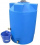The block-shaped tank is 2.5 m long, 100 cm wide and 12 dm high. In how many minutes will it be filled with water to two thirds if 40 liters of water flow into the tank per minute?
• Fire tankHow deep is the fire tank with the dimensions of the bottom 7m and 12m, when filled with 420 m3 of water?
• HectolitersHow many hectoliters of water fits into cuboid tank with dimensions of a = 3.5 m b = 2.5 m c = 1.4 m?
• Tank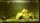The tank bottom has dimensions of 1.5 m and 3 2/6 m. The tank is 459.1 hl of water. How high is the water surface?
• The rainwaterThe rainwater container has the shape of a block whose bottom has dimensions of 4.5 m and 3.5 m. It is partly filled with water. What is the level if there is 189 hl of water in it?
• Pool 3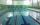How long will fill pool cuboid shape (8m 6m 1.5m) when flows 15 liters/s?
• Rain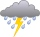Garden shape of a rectangle measuring 15 m and 20 m rained water up to 3 mm. How many liters of water rained in the garden?
• Swimming pool 4The pool shaped cuboid measuring 12.5 m × 640 cm at the bottom is 960hl water. To what height in meters reaches the water level?
• Tank full2 hl of water were poured into a block-shaped tank with dimensions of 450 mm and 600 mm and a height of 900 mm. How many liters of water need to be added to keep the tank full?
• TanksThe fire tank has a cuboid shape with a rectangular floor measuring 13.7 m × 9.8 m. Water depth is 2.4 m. Water was pumped from the tank into barrels with a capacity of 2.7 hl. How many barrels were used if the water level in the tank fallen 5 cm? W
• The tankThe tank has 1320 liters of water. The tank has the shape of a prism, its base is an rectangle with sides a = 0,6 m and b = 1,5 m. How high does the water level reach in the tank?